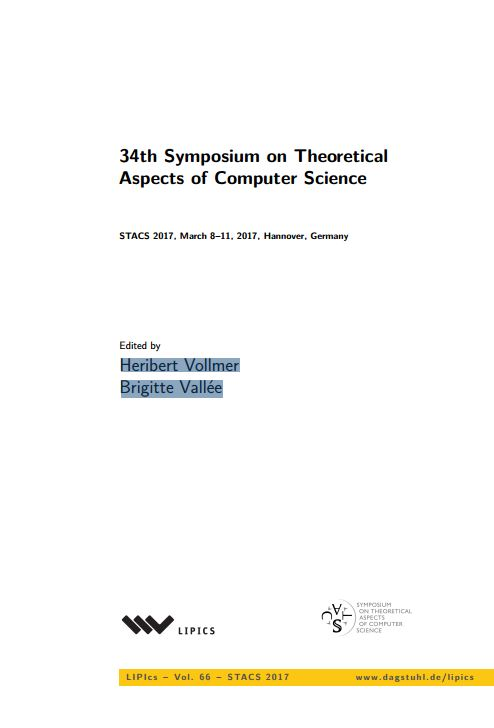• A
• A
• A
• АБB
• АБB
• АБB
• А
• А
• А
• А
• А
Обычная версия сайта

## Computing majority by constant depth majority circuits with low fan-in gates

P. 1-14.
Kulikov A., Podolskii V. V.

We study the following computational problem: for which values of k, the majority of n bits MAJn can be computed with a depth two formula whose each gate computes a majority function of at most k bits? The corresponding computational model is denoted by MAJk o MAJk. We observe that the minimum value of k for which there exists a MAJk o MAJk circuit that has high correlation with the majority of n bits is equal to Θ(n1/2). We then show that for a randomized MAJk o MAJk circuit computing the majority of n input bits with high probability for every input, the minimum value of k is equal to n2/3+o(1). We show a worst case lower bound: if a MAJk o MAJk circuit computes the majority of n bits correctly on all inputs, then k ≥ n13/19+o(1). This lower bound exceeds the optimal value for randomized circuits and thus is unreachable for pure randomized techniques. For depth 3 circuits we show that a circuit with k = O(n2/3) can compute MAJn correctly on all inputs.

### В книгеТ. 66. Лейпциг: Schloss Dagstuhl--Leibniz-Zentrum fuer Informatik, 2017.